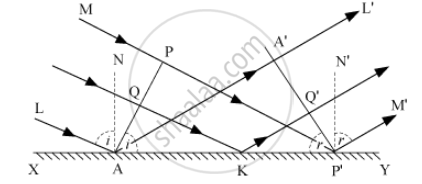Department of Pre-University Education, KarnatakaPUC Karnataka Science Class 12

# Use Huygens' principle to verify the laws of refraction. - Physics

Use Huygens' principle to verify the laws of refraction.

#### Solution

Huygens' Principle to Verify the Laws of Refraction :Consider any point Q on the incident wavefront PA.

When the disturbance from P on incident wavefront reaches point P', the disturbance from point Q reaches Q'.

If c is velocity of light, then time taken by light to go from point Q to Q'(via point K) is given by,

t=(QK)/c+(KQ')/c....(i)

In right-angled ΔAQK,

∠QAK = i

∴ QK = AK sin i

In right-angled ΔKQ'P'

∠Q'P'K=r

∴ KQ'=KP'sin r

Substituting these values in equation (i),

t=(AKsini)/c+(KP'sinr)/c

t=(AKsini+KP'sinr)/c

t=(AKsini+(AP'-AK)sinr)/c [∵ KP'=AP'-AK]

t=(AP'sinr+AK(sini-sinr))/c   .....(ii)

The rays from different points on incident wavefront will take the same time to reach the corresponding points on the reflected wavefront, if ‘t’ given by equation (ii) is independent of AK.

∴ AK (sin i − sin r) = 0

sin i − sin r = 0

sin i = sin r

i = r

i.e., the angle of incidence is equal to the angle of reflection.

Also, the incident ray (LA or MP'), reflected ray (AA'L' or P'M'), and the normal (AN) − all lie in the same plane.

Concept: Huygens Principle
Is there an error in this question or solution?